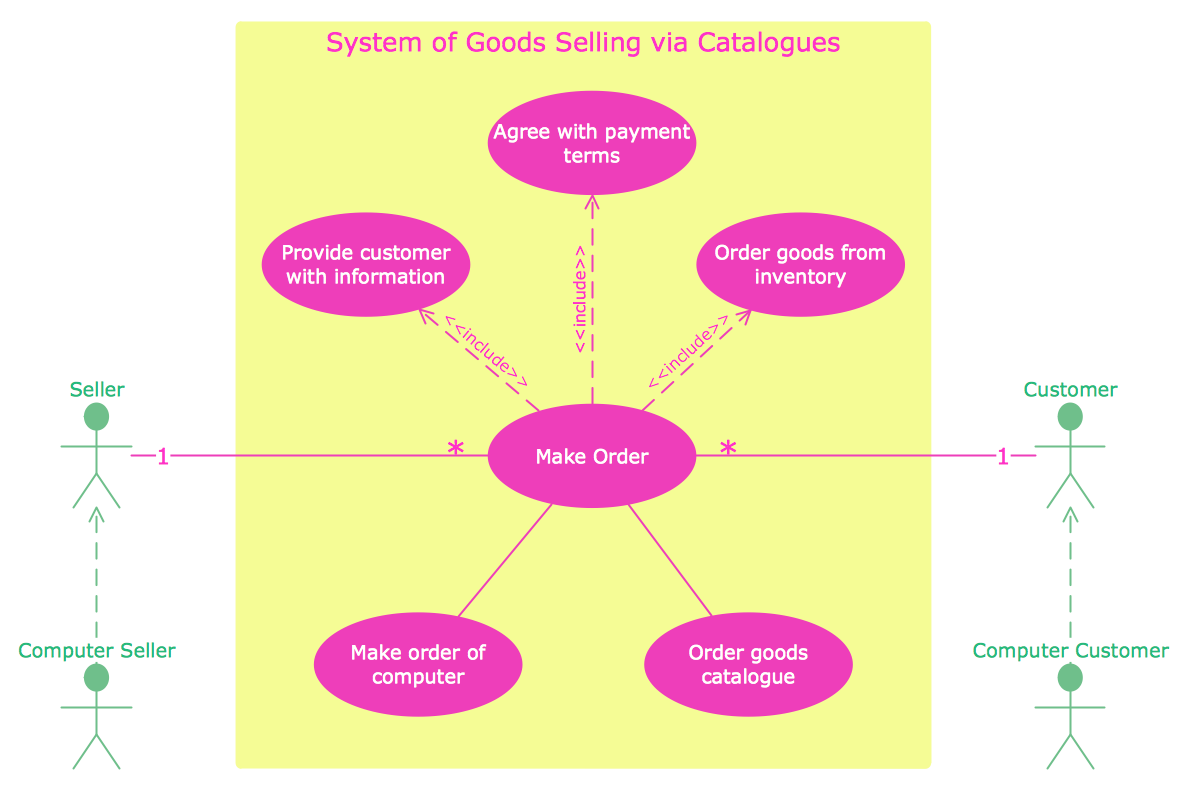This site uses cookies. By continuing to browse the ConceptDraw site you are agreeing to our Use of Site Cookies.
ConceptDraw Samples | Software development — Rapid UML

# Rapid UML

The UML 2.4 diagrams are created using ConceptDraw DIAGRAM diagramming and vector drawing software enhanced with Rapid UML solution from ConceptDraw Solution Park.

ConceptDraw DIAGRAM provides export of vector graphic multipage documents into multiple file formats: vector graphics (SVG, EMF, EPS), bitmap graphics (PNG, JPEG, GIF, BMP, TIFF), web documents (HTML, PDF), PowerPoint presentations (PPT), Adobe Flash (SWF).

## Tutorials and Solutions:

Video Tutorials: ConceptDraw Solution Park

Solutions: Rapid UML for ConceptDraw DIAGRAM

## Sample 1: UML Sequence Diagram — Checking Process

Rapid UML sample: UML sequence diagram — Checking process.

This example is created using ConceptDraw DIAGRAM diagramming software enhanced with Rapid UML solution from ConceptDraw Solution Park.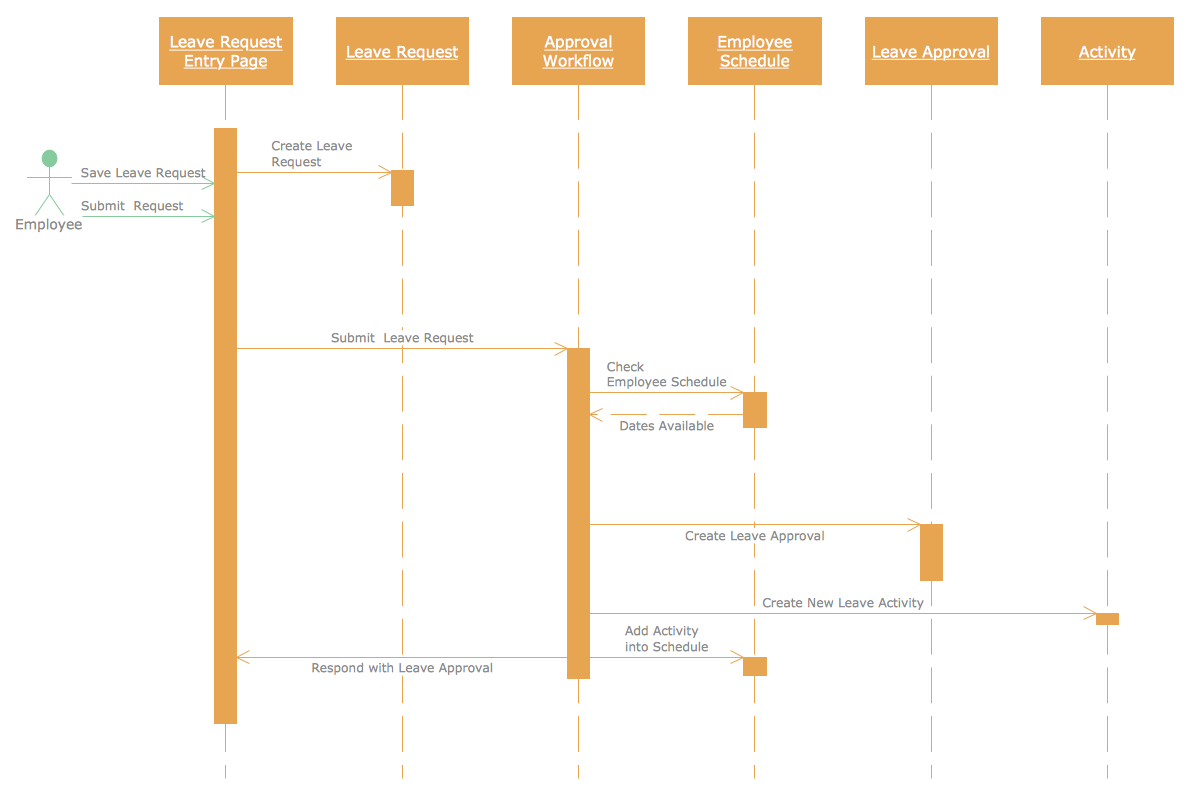## Sample 2: UML Activity Diagram — Payment Process

Rapid UML sample: UML activity diagram — Payment process.

This example is created using ConceptDraw DIAGRAM diagramming software enhanced with Rapid UML solution from ConceptDraw Solution Park.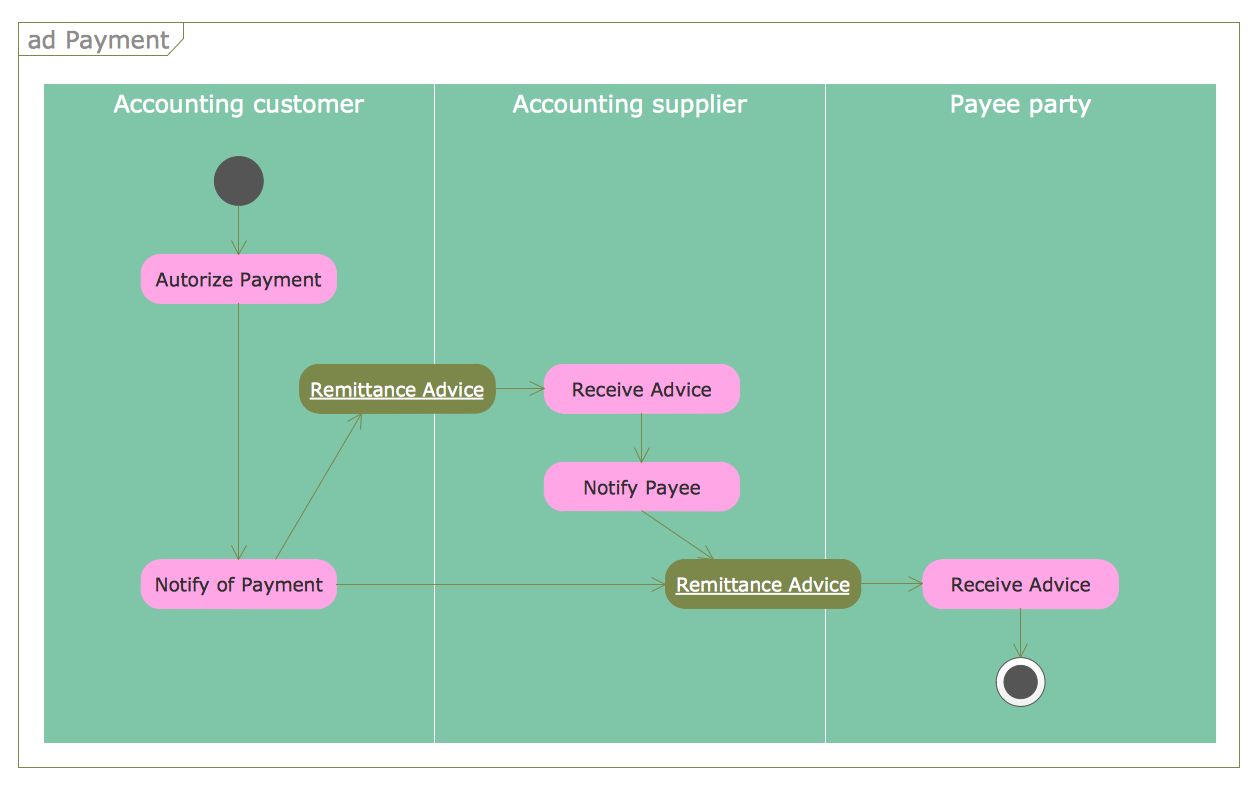## Sample 3: UML Deployment Diagram

Rapid UML sample: UML deployment diagram.

This example is created using ConceptDraw DIAGRAM diagramming software enhanced with Rapid UML solution from ConceptDraw Solution Park.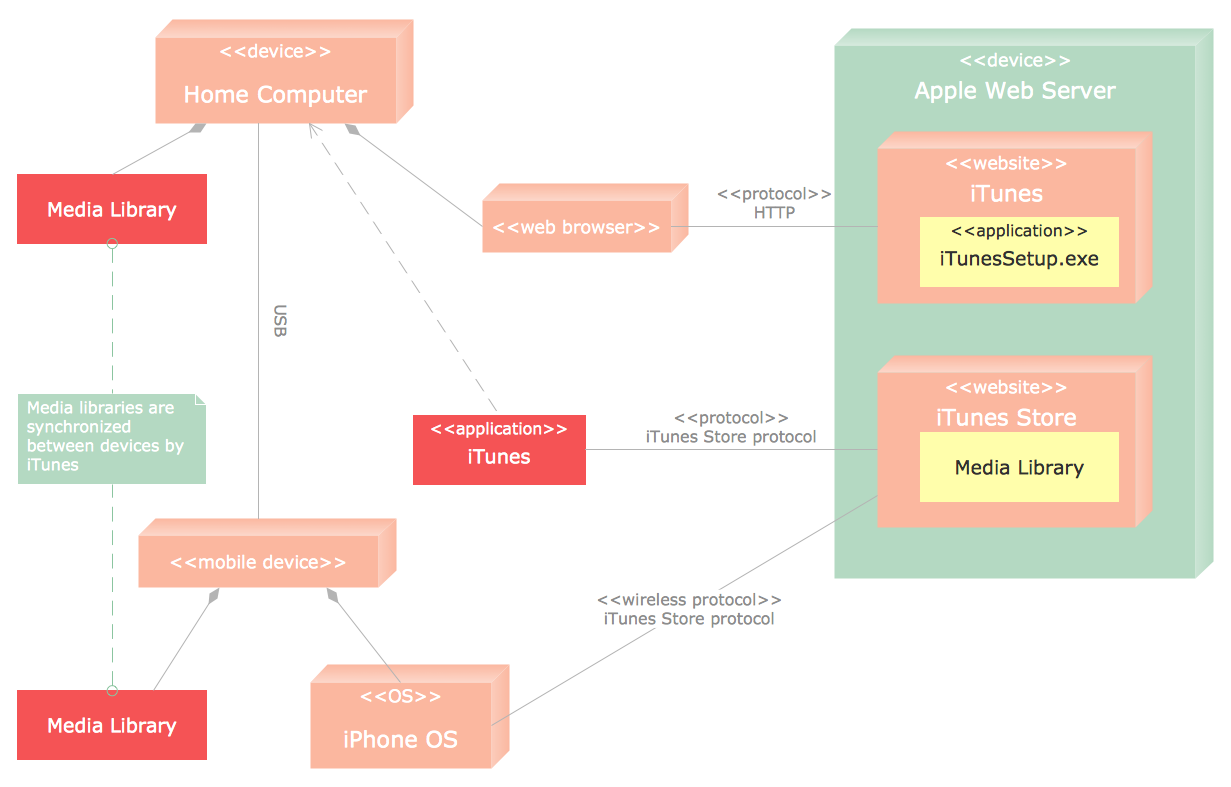## Sample 4: UML Class Diagram

Rapid UML sample: UML class diagram.

This example is created using ConceptDraw DIAGRAM diagramming software enhanced with Rapid UML solution from ConceptDraw Solution Park.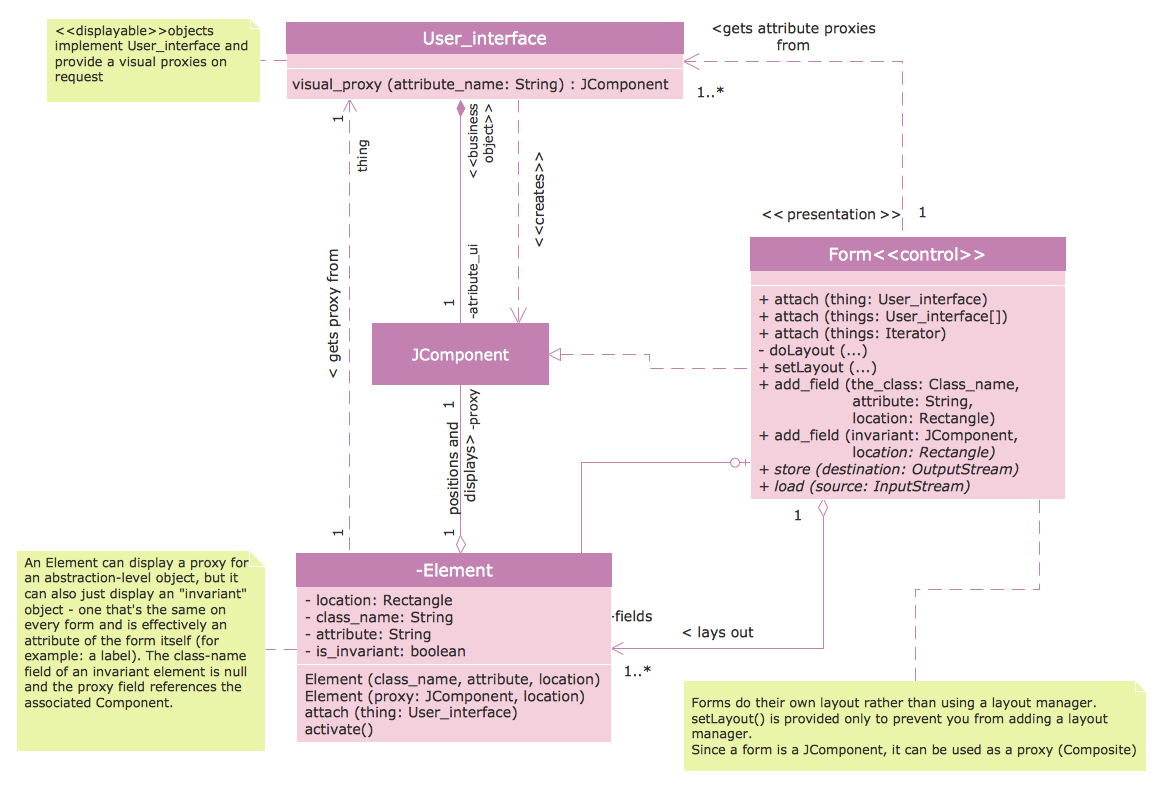## Sample 5: UML Use Case Diagram — Banking System

Rapid UML sample: UML use case diagram.

This example is created using ConceptDraw DIAGRAM diagramming software enhanced with Rapid UML solution from ConceptDraw Solution Park.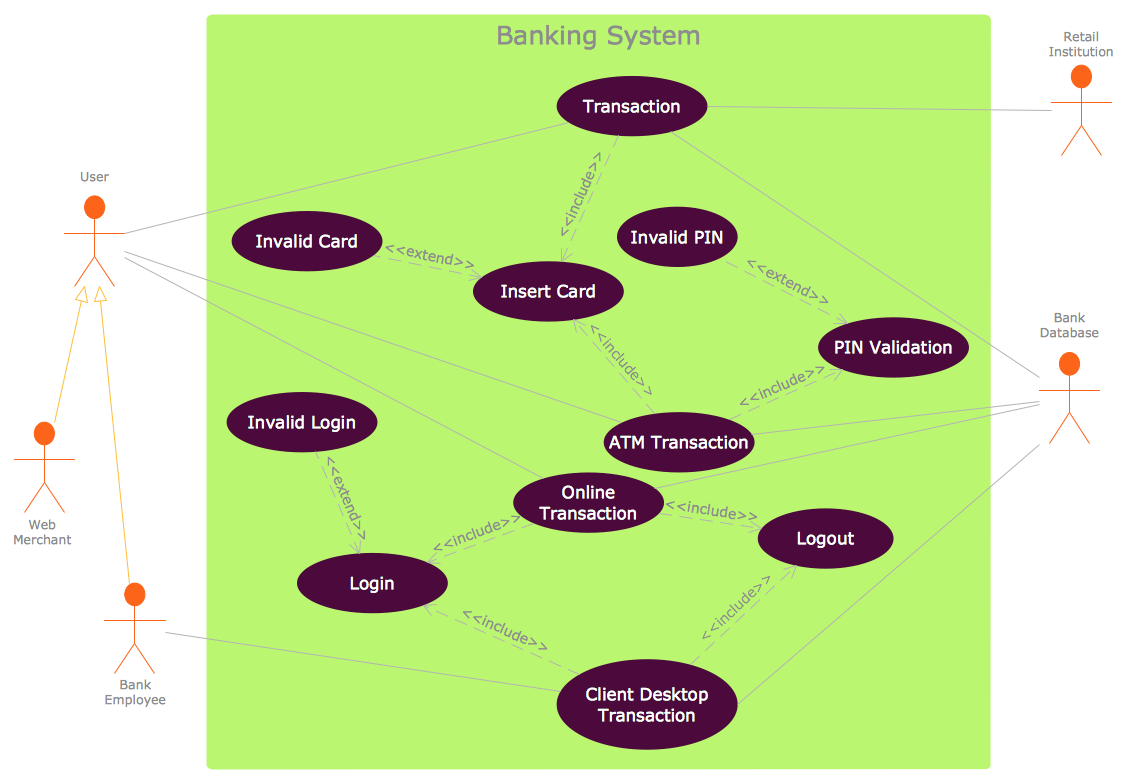## Sample 6: UML Activity Diagram — Ticket Processing System

Rapid UML sample: UML activity diagram — Ticket processing system.

This example is created using ConceptDraw DIAGRAM diagramming software enhanced with Rapid UML solution from ConceptDraw Solution Park.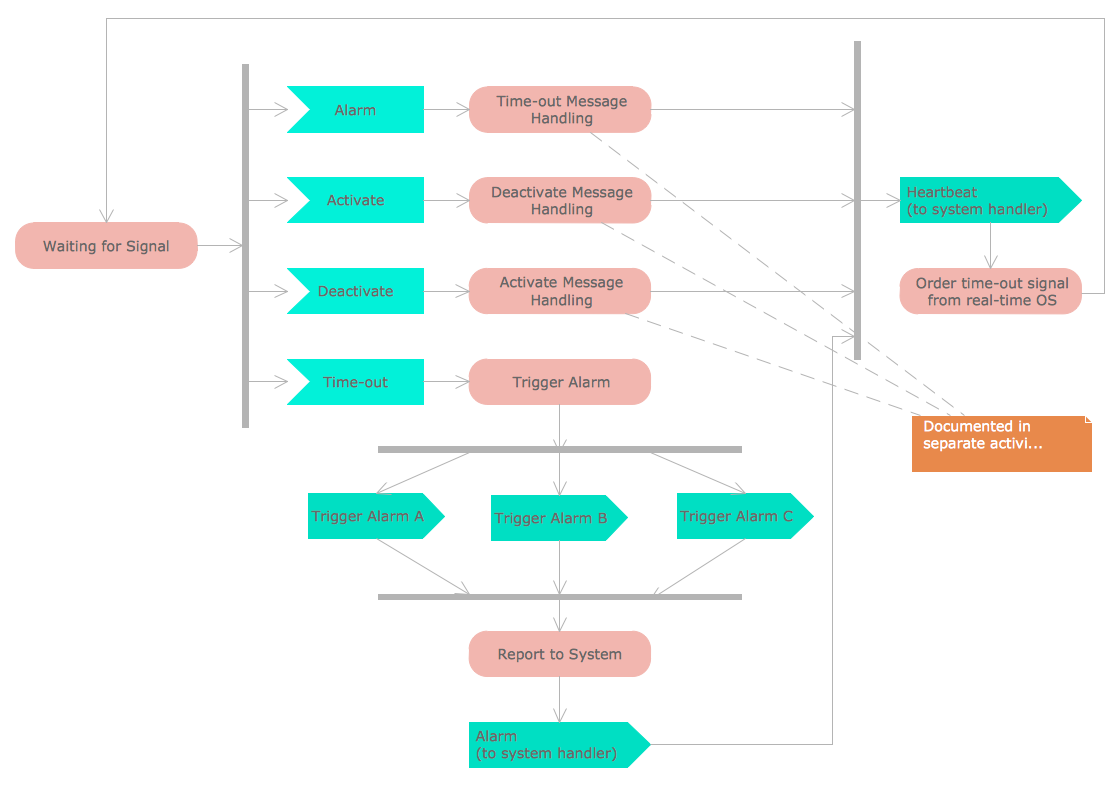## Sample 7: UML Activity Diagram — Cash Withdrawal from ATM

Rapid UML sample: UML activity diagram — Cash withdrawal from ATM.

This example is created using ConceptDraw DIAGRAM diagramming software enhanced with Rapid UML solution from ConceptDraw Solution Park.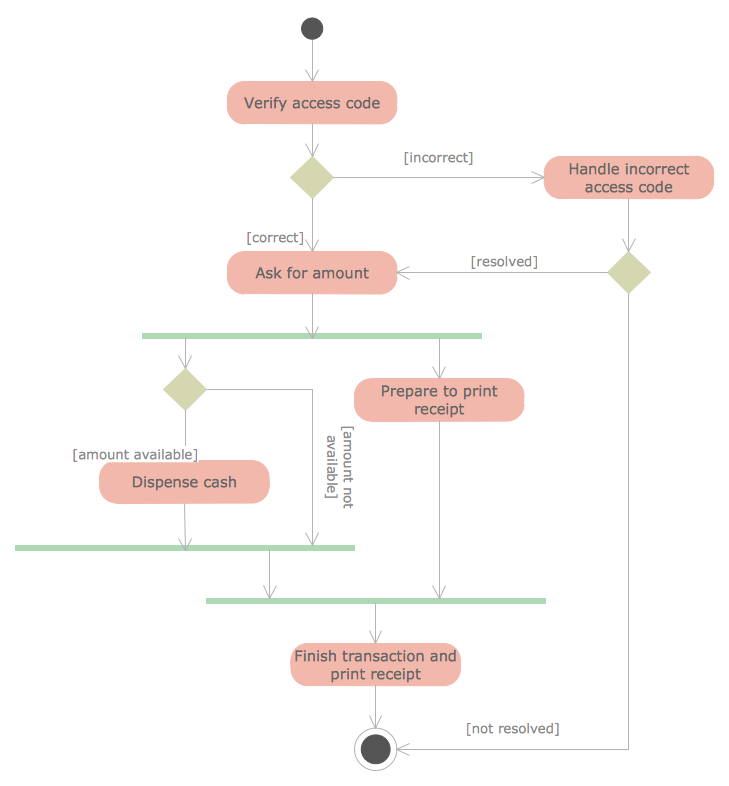## Sample 8: UML Activity Diagram — Catalogue Creation Process

Rapid UML sample: UML activity diagram — Catalogue creation process.

This example is created using ConceptDraw DIAGRAM diagramming software enhanced with Rapid UML solution from ConceptDraw Solution Park.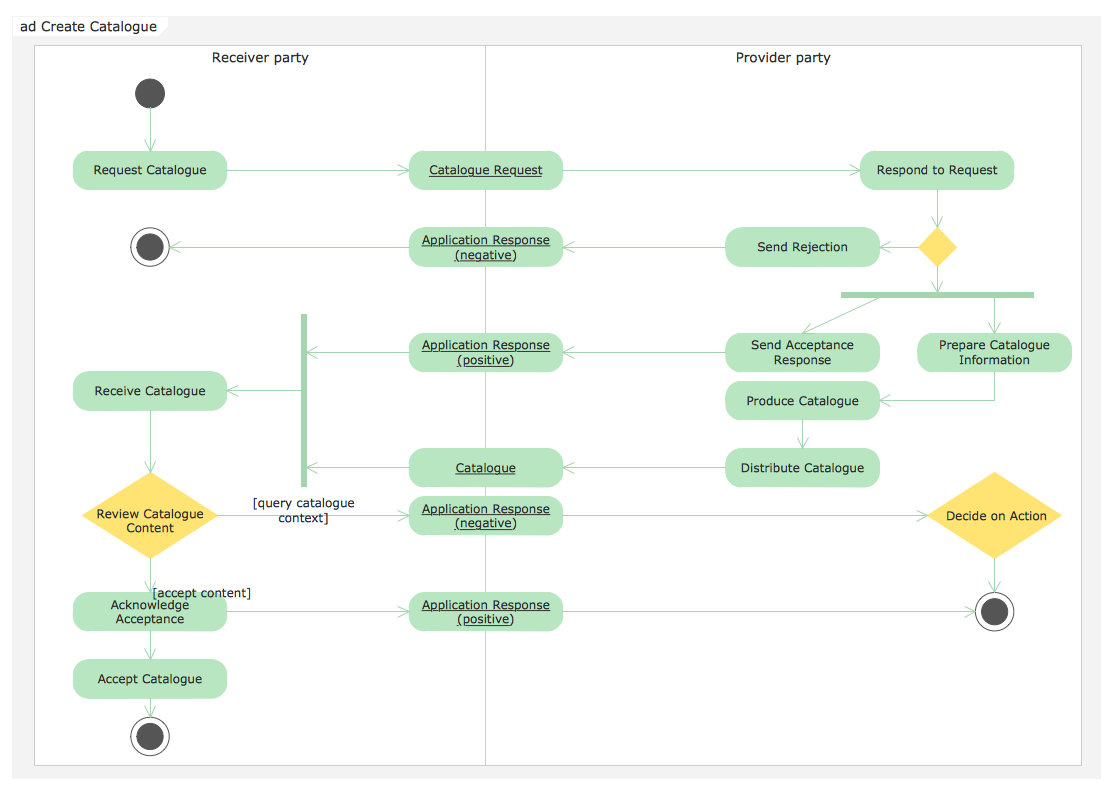## Sample 9: UML Activity Diagram — Deposit Slip Processing

Rapid UML sample: UML activity diagram — Deposit slip processing.

This example is created using ConceptDraw DIAGRAM diagramming software enhanced with Rapid UML solution from ConceptDraw Solution Park.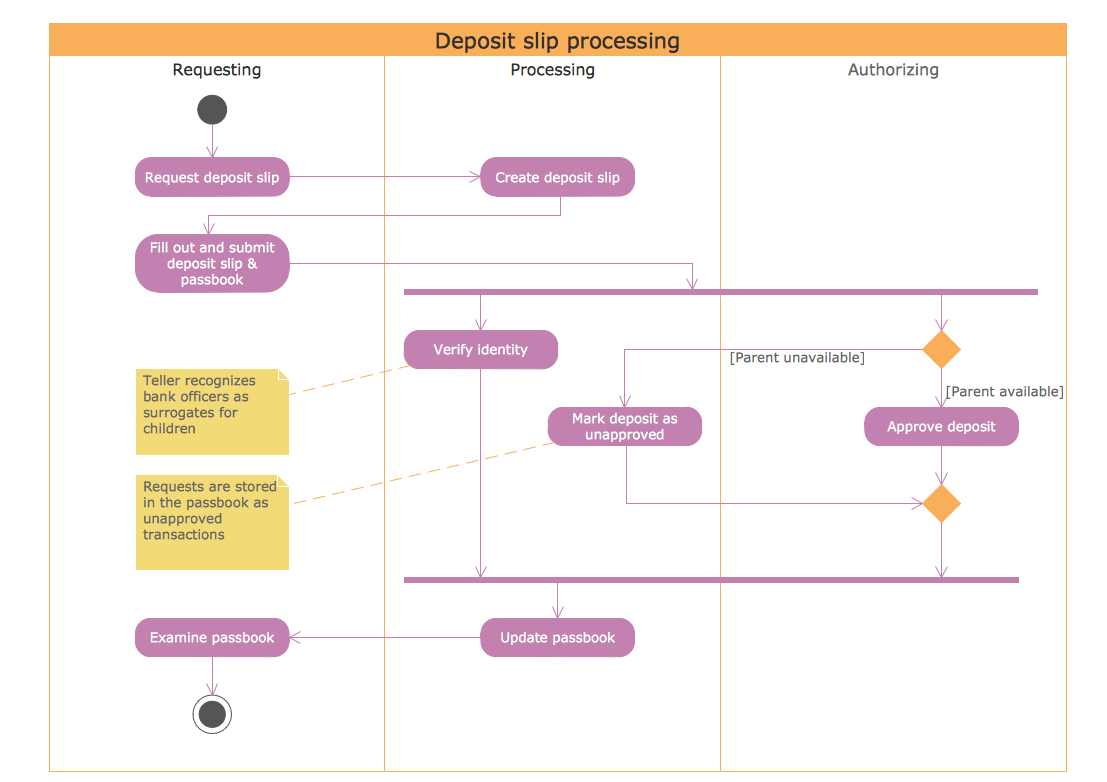## Sample 10: UML Activity Diagram — Servlet Container

Rapid UML sample: UML activity diagram — Servlet container.

This example is created using ConceptDraw DIAGRAM diagramming software enhanced with Rapid UML solution from ConceptDraw Solution Park.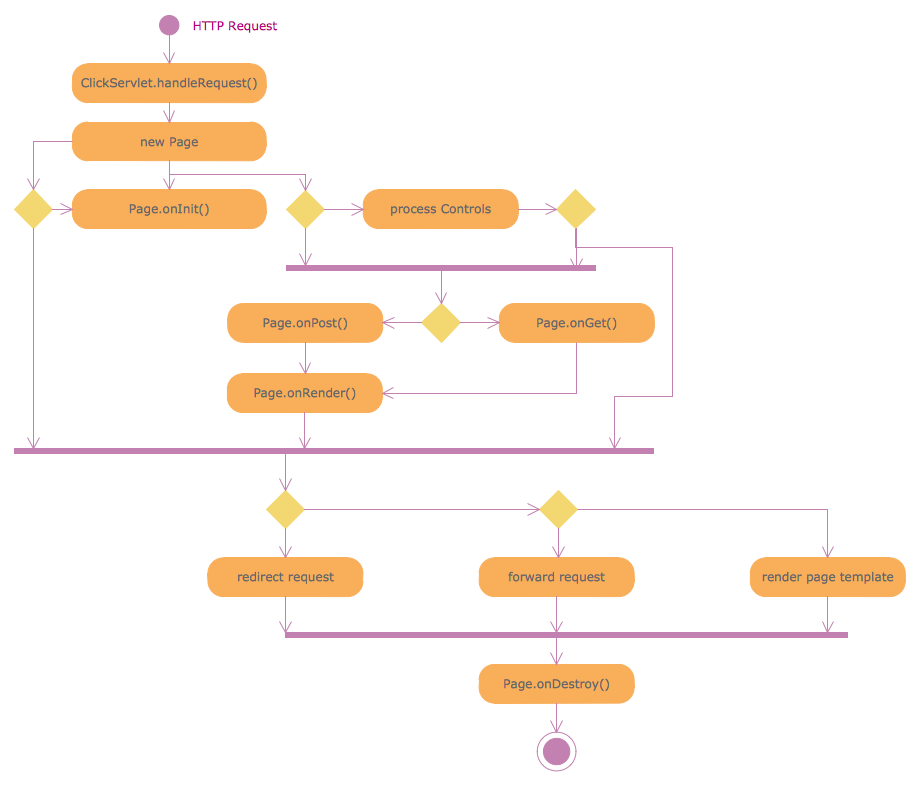## Sample 11: UML Activity Diagram — Ticket Processing System

Rapid UML sample: UML activity diagram — Ticket processing system.

This example is created using ConceptDraw DIAGRAM diagramming software enhanced with Rapid UML solution from ConceptDraw Solution Park.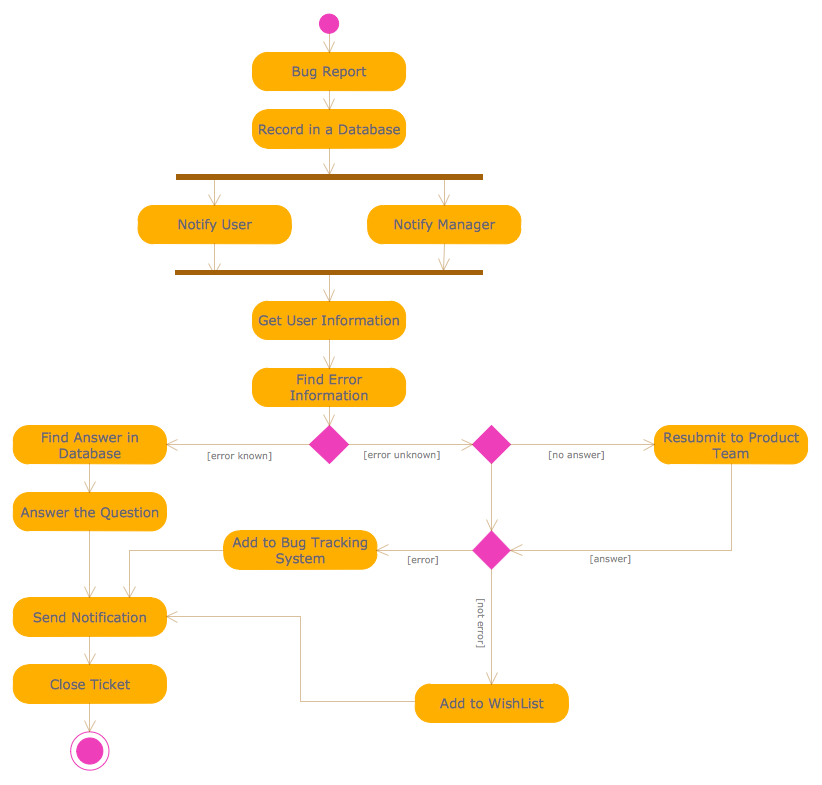## Sample 12: UML Activity Diagram — Snap in Process

Rapid UML sample: UML activity diagram — Snap in process.

This example is created using ConceptDraw DIAGRAM diagramming software enhanced with Rapid UML solution from ConceptDraw Solution Park.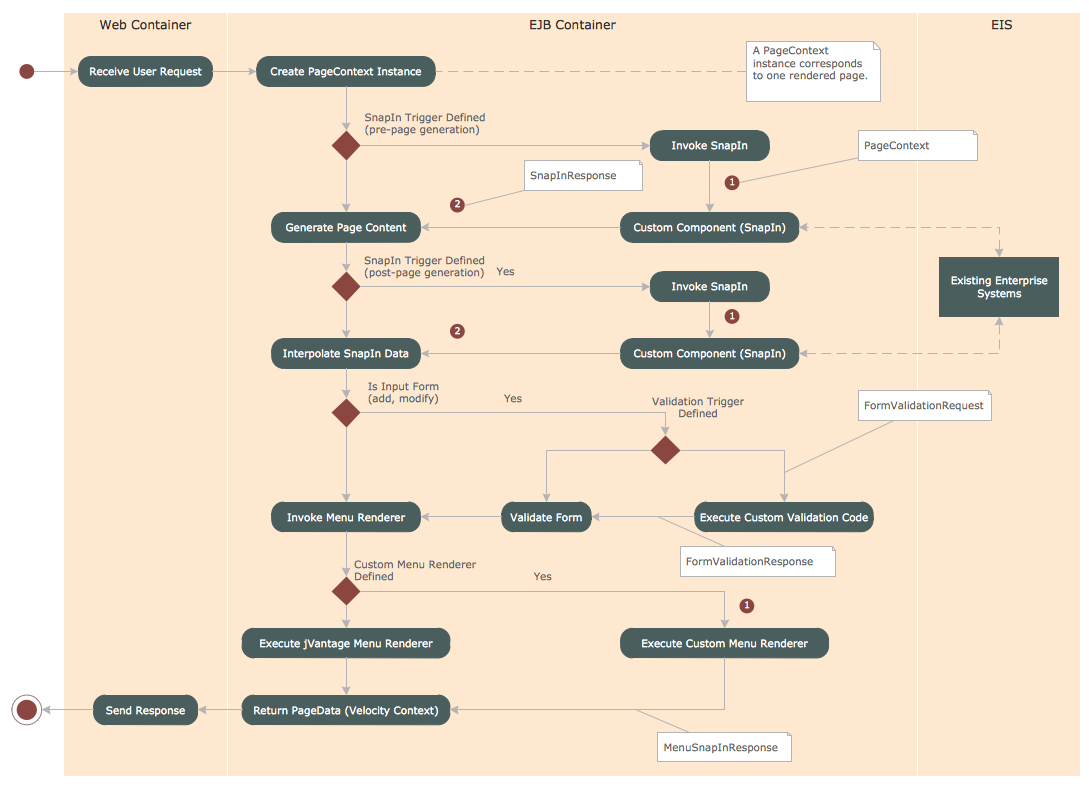## Sample 13: UML Activity Diagram — User Registration

Rapid UML sample: UML activity diagram — User registration.

This example is created using ConceptDraw DIAGRAM diagramming software enhanced with Rapid UML solution from ConceptDraw Solution Park.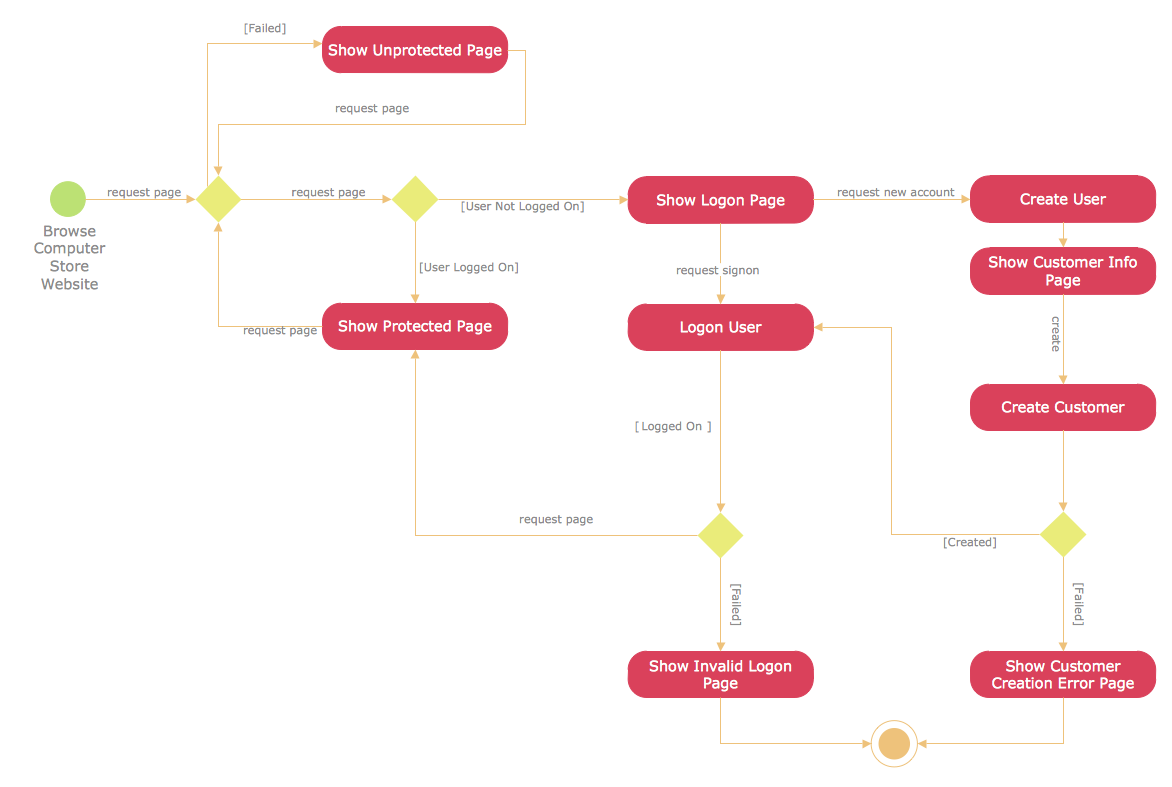## Sample 14: UML Class Diagram — Metadata Information Model

Rapid UML sample: UML class diagram — Metadata information model.

This example is created using ConceptDraw DIAGRAM diagramming software enhanced with Rapid UML solution from ConceptDraw Solution Park.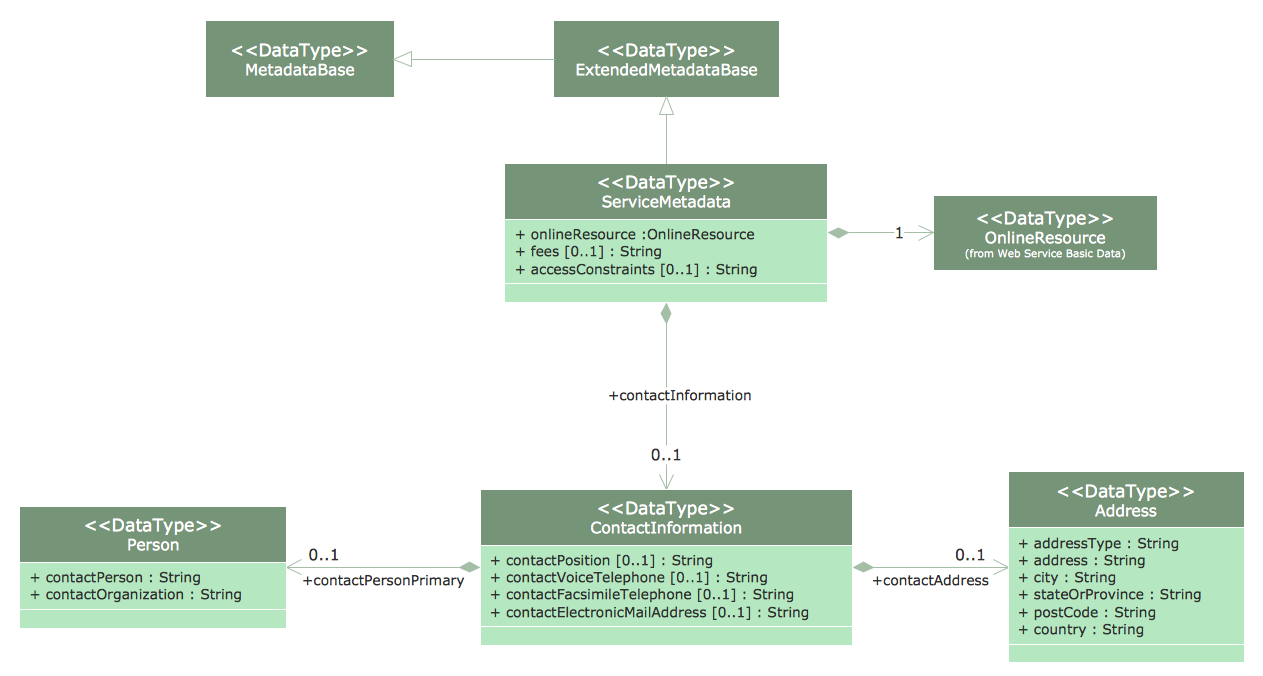## Sample 15: UML Collaboration Diagram — Ticket Processing System

Rapid UML sample: UML collaboration diagram — Ticket processing system.

This example is created using ConceptDraw DIAGRAM diagramming software enhanced with Rapid UML solution from ConceptDraw Solution Park.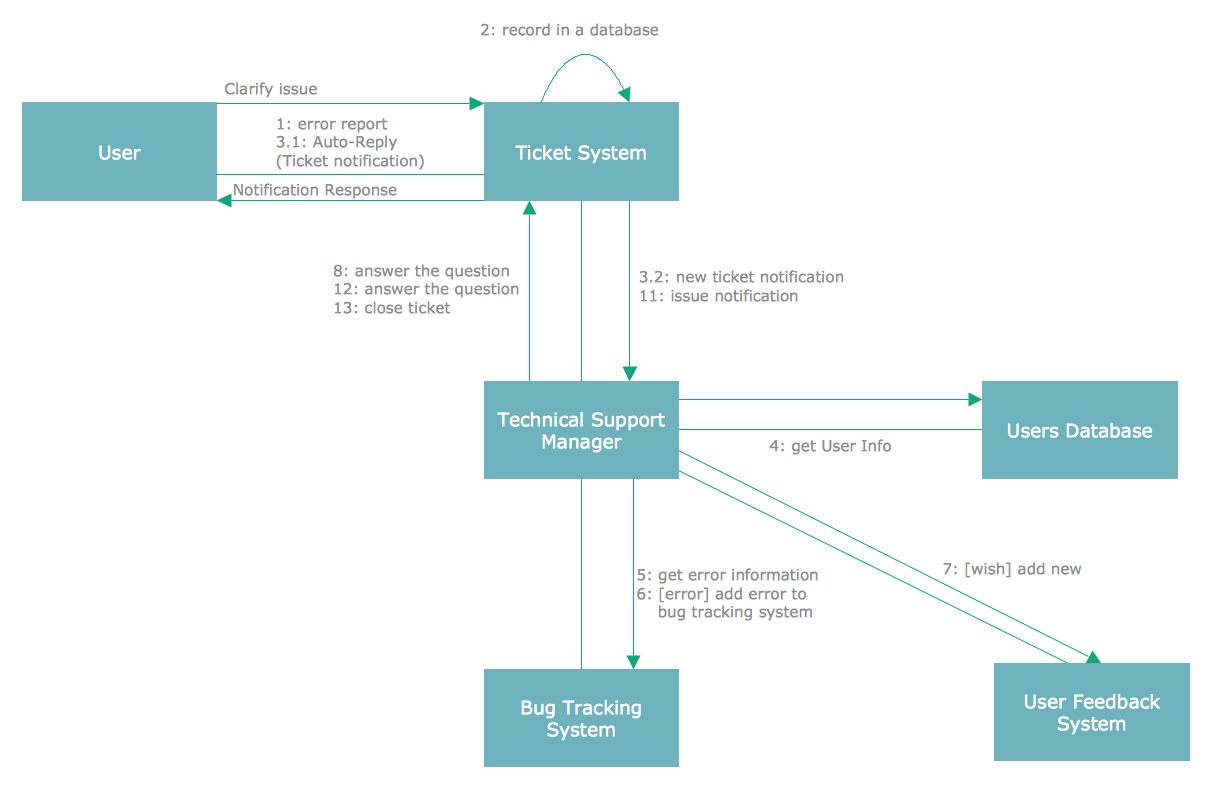## Sample 16: UML Communication Diagram — Client Server Access

Rapid UML sample: UML communication diagram — Client server access.

This example is created using ConceptDraw DIAGRAM diagramming software enhanced with Rapid UML solution from ConceptDraw Solution Park.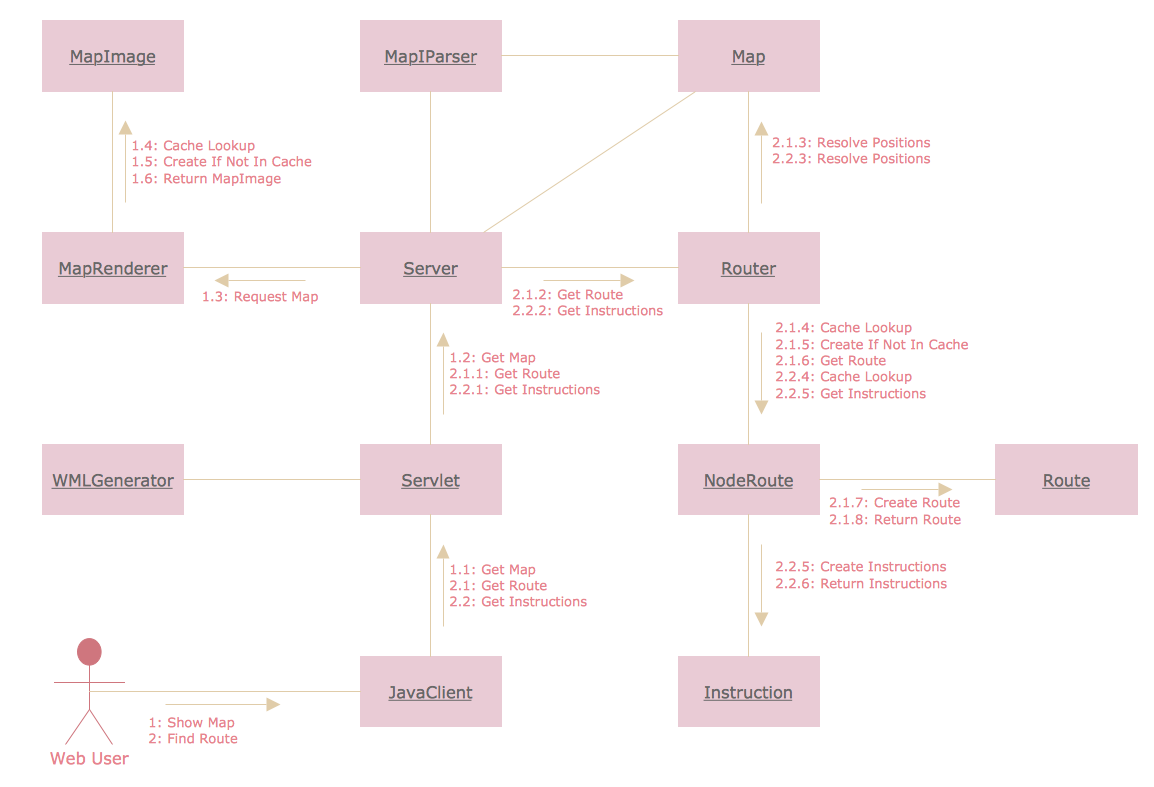## Sample 17: UML Component Diagram — Credit Card Agency

Rapid UML sample: UML component diagram — Credit card agency.

This example is created using ConceptDraw DIAGRAM diagramming software enhanced with Rapid UML solution from ConceptDraw Solution Park.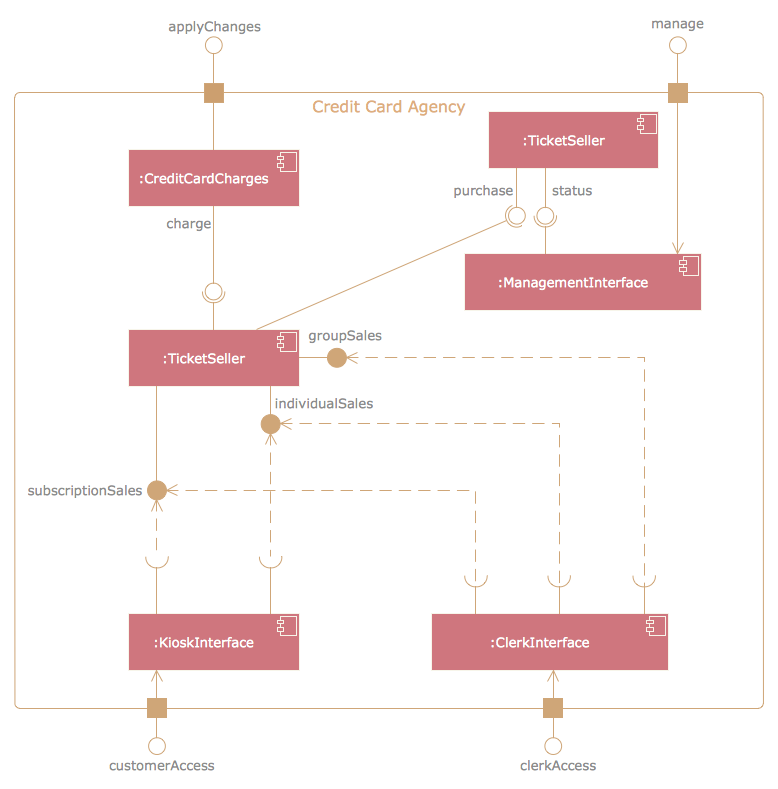## Sample 18: UML Component Diagram — Start Server

Rapid UML sample: UML component diagram — Start server.

This example is created using ConceptDraw DIAGRAM diagramming software enhanced with Rapid UML solution from ConceptDraw Solution Park.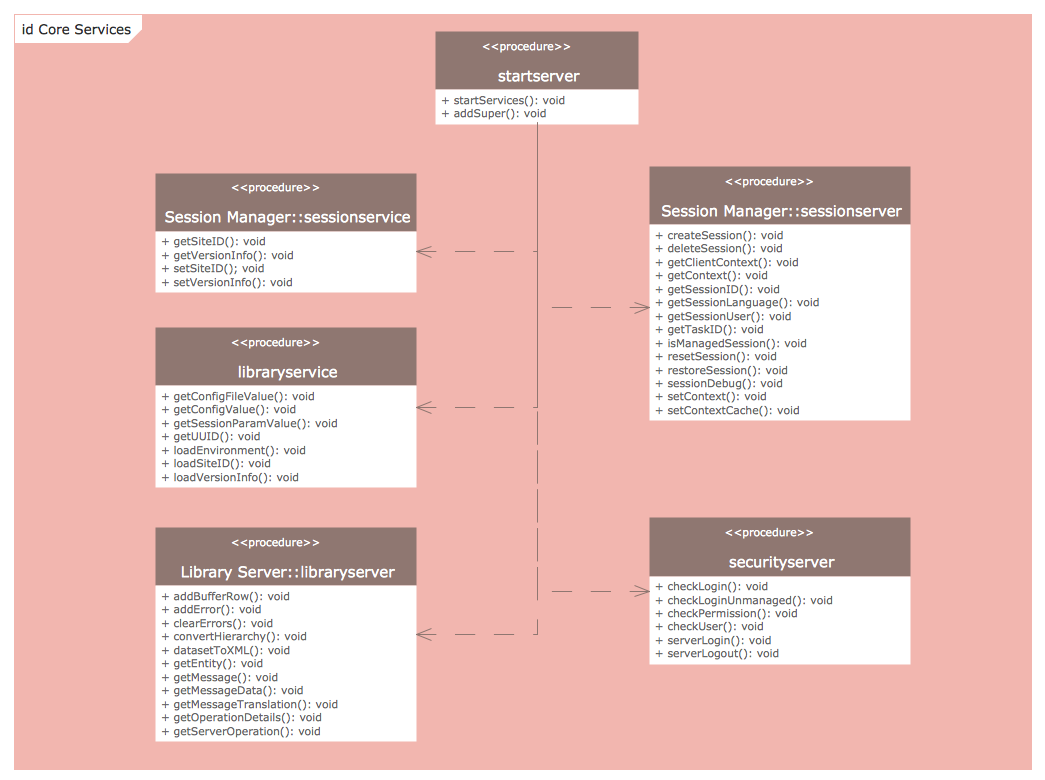## Sample 19: UML Component Diagram Template

Rapid UML sample: UML component diagram template.

This example is created using ConceptDraw DIAGRAM diagramming software enhanced with Rapid UML solution from ConceptDraw Solution Park.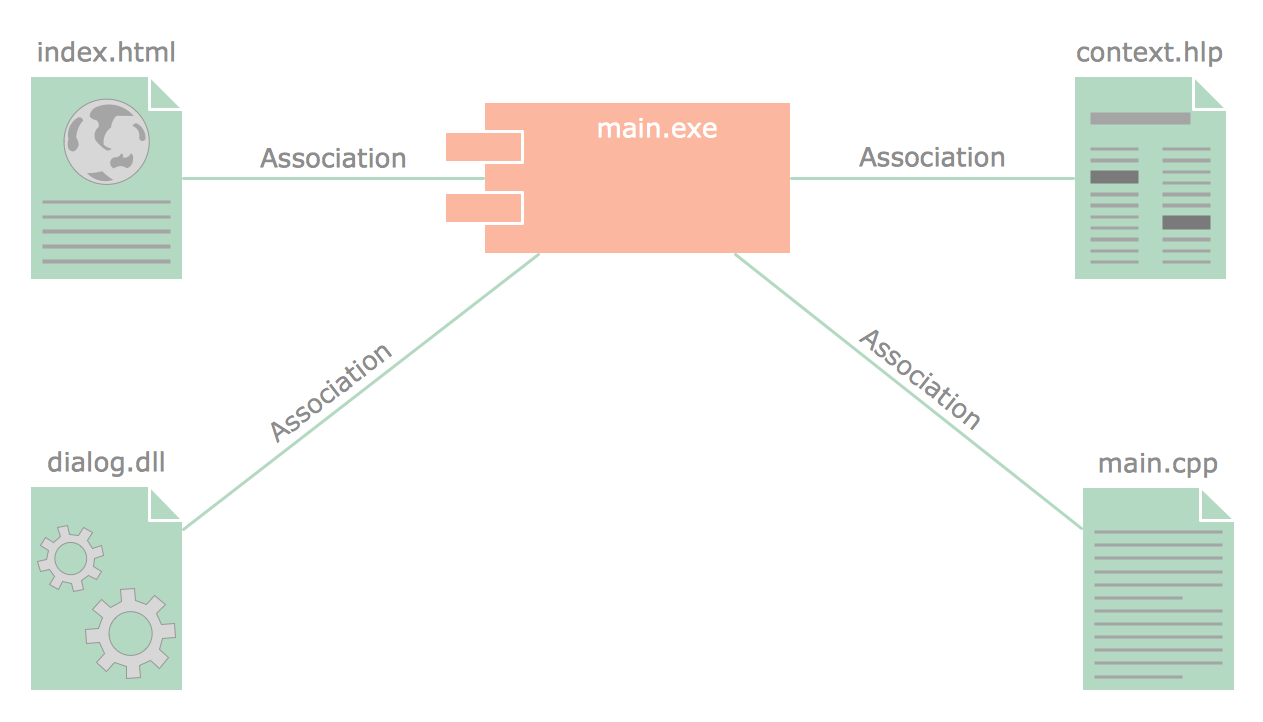## Sample 20: UML Composite Structure Diagram — Drive a Taxi

Rapid UML sample: UML composite structure diagram — Drive a taxi.

This example is created using ConceptDraw DIAGRAM diagramming software enhanced with Rapid UML solution from ConceptDraw Solution Park.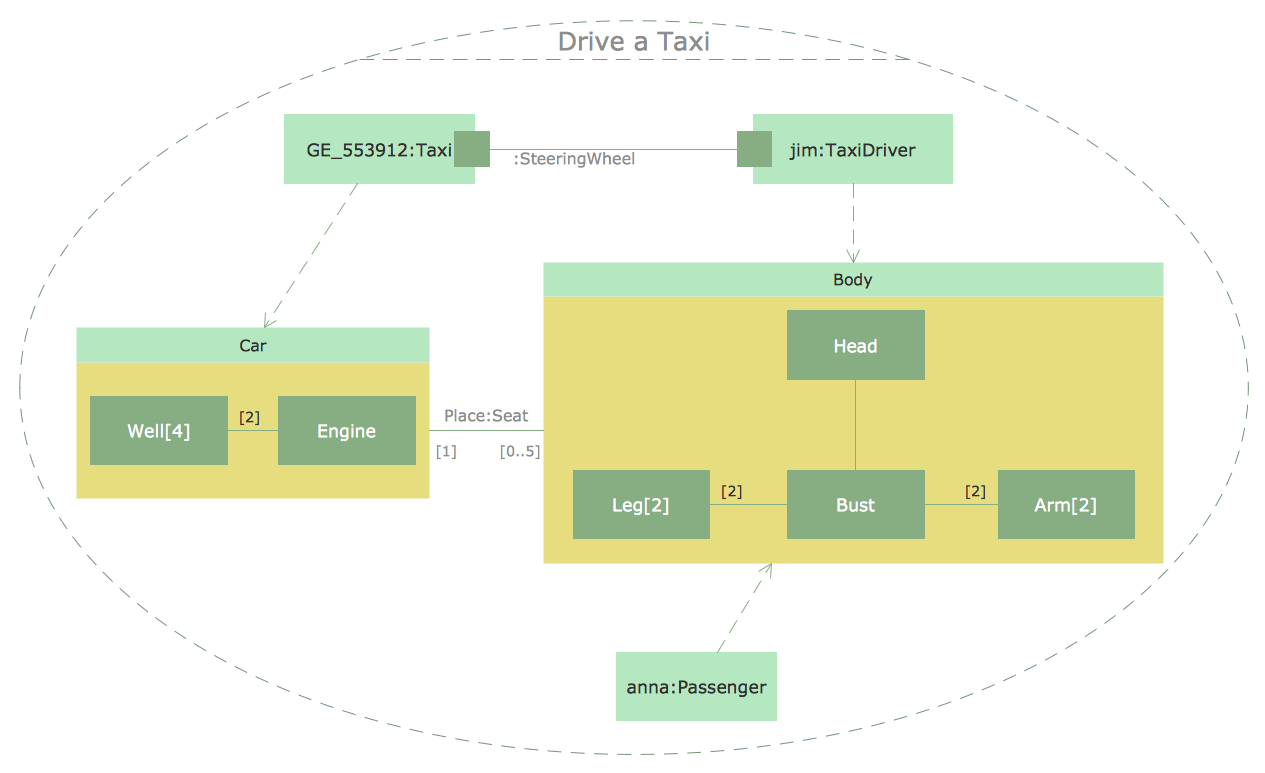## Sample 21: UML Composite Structure Diagram — Sale Process

Rapid UML sample: UML composite structure diagram — Sale process.

This example is created using ConceptDraw DIAGRAM diagramming software enhanced with Rapid UML solution from ConceptDraw Solution Park.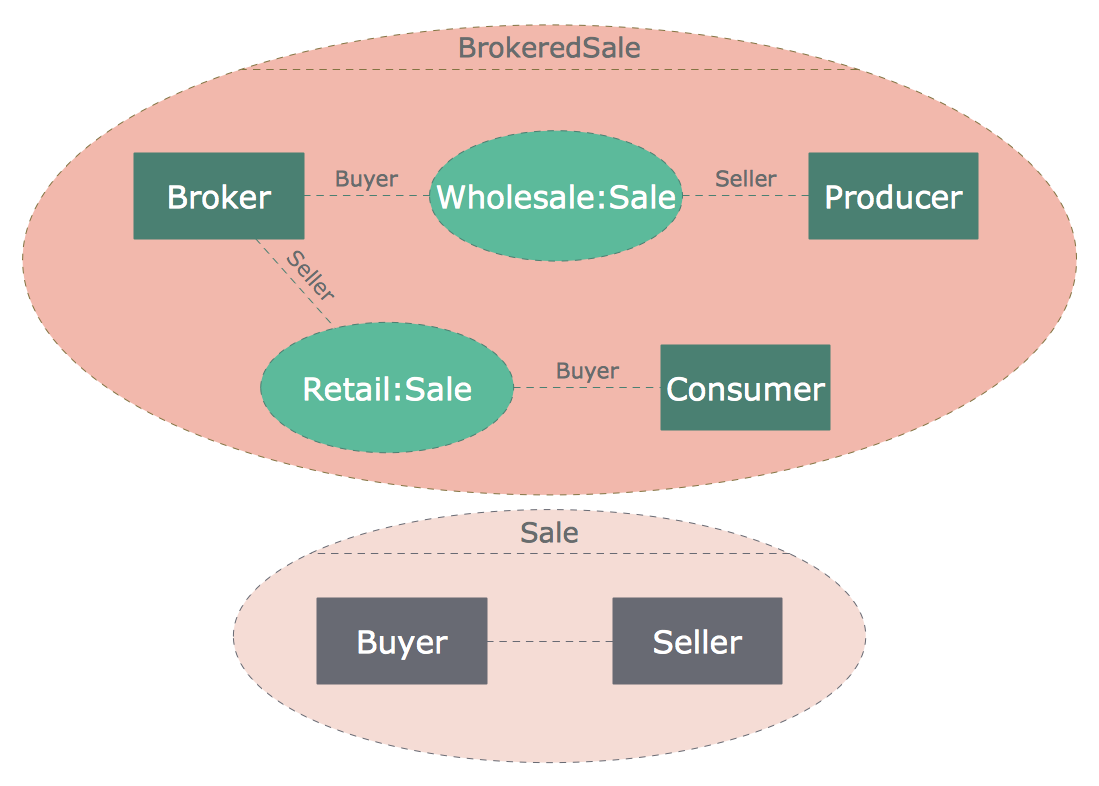## Sample 22: UML Deployment Diagram — Real Estate Transactions

Rapid UML sample: UML deployment diagram — Real estate transactions.

This example is created using ConceptDraw DIAGRAM diagramming software enhanced with Rapid UML solution from ConceptDraw Solution Park.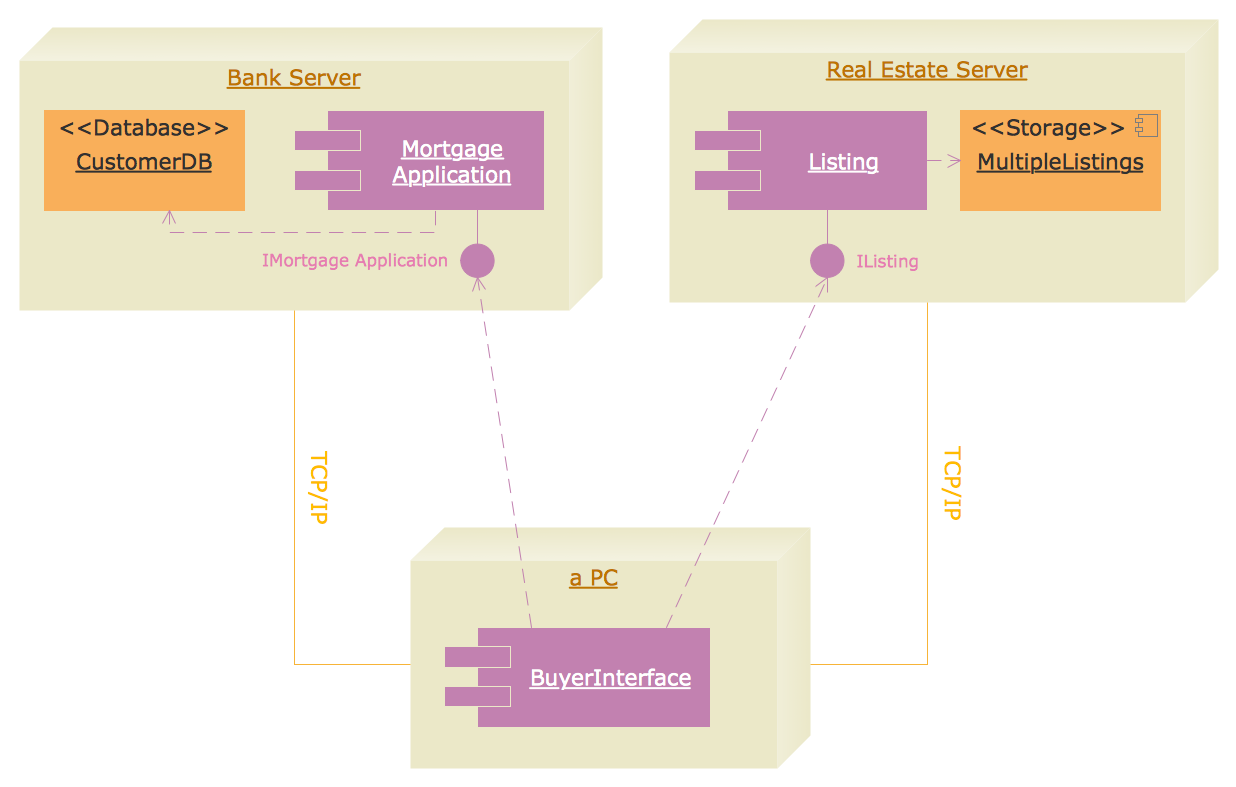## Sample 23: UML Deployment Diagram

Rapid UML sample: UML Deployment Diagram.

This example is created using ConceptDraw DIAGRAM diagramming software enhanced with Rapid UML solution from ConceptDraw Solution Park.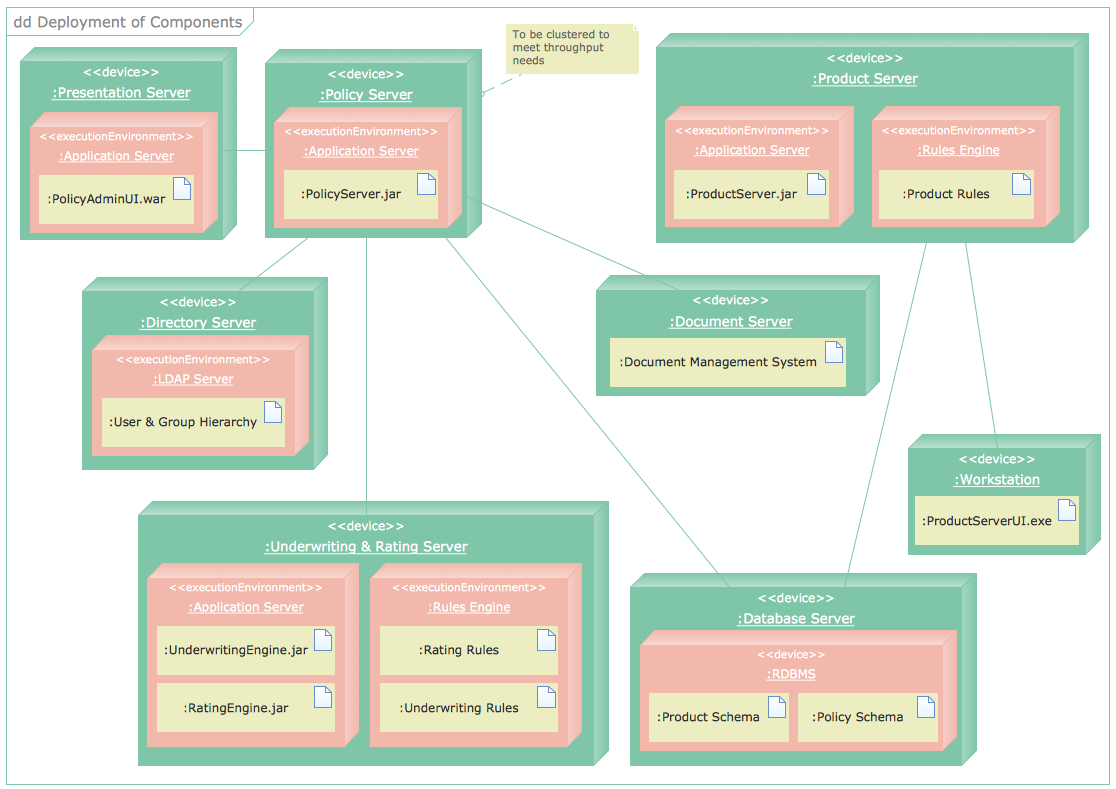## Sample 24: UML Interaction Overview Diagram

Rapid UML sample: UML interaction overview diagram.

This example is created using ConceptDraw DIAGRAM diagramming software enhanced with Rapid UML solution from ConceptDraw Solution Park.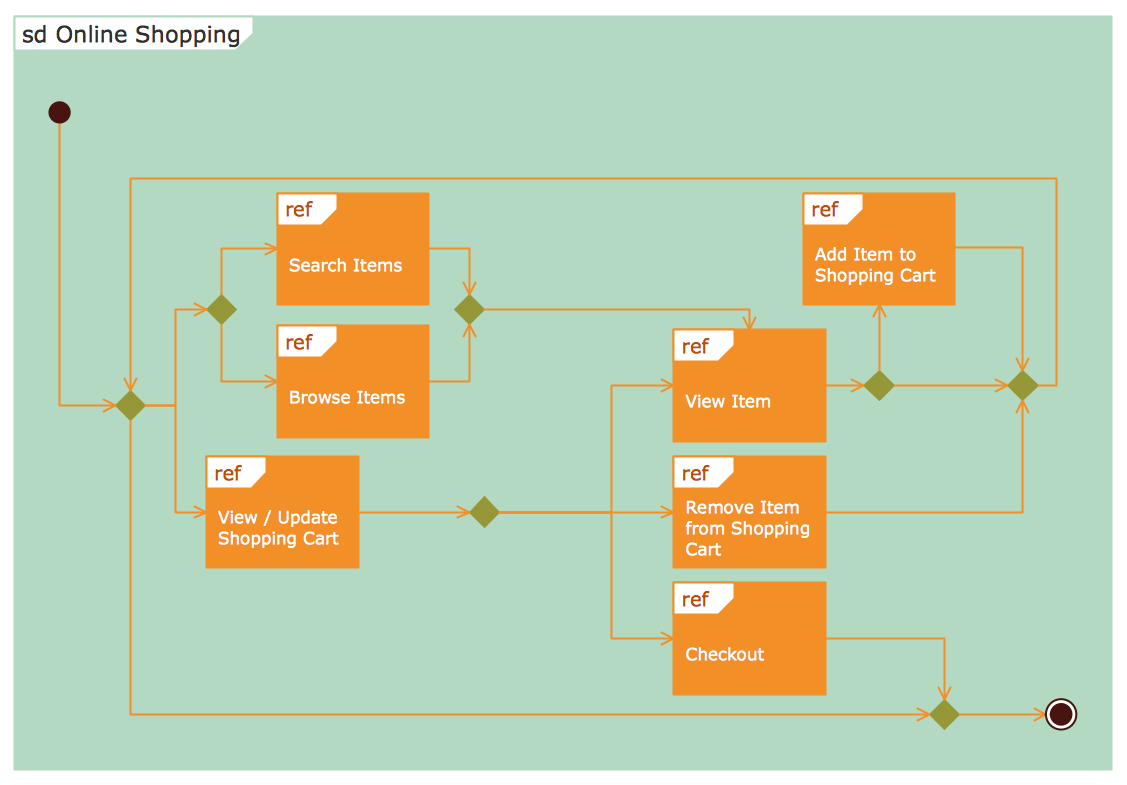## Sample 25: UML Object Diagram — Estimating Scenario

Rapid UML sample: UML object diagram — Estimating scenario.

This example is created using ConceptDraw DIAGRAM diagramming software enhanced with Rapid UML solution from ConceptDraw Solution Park.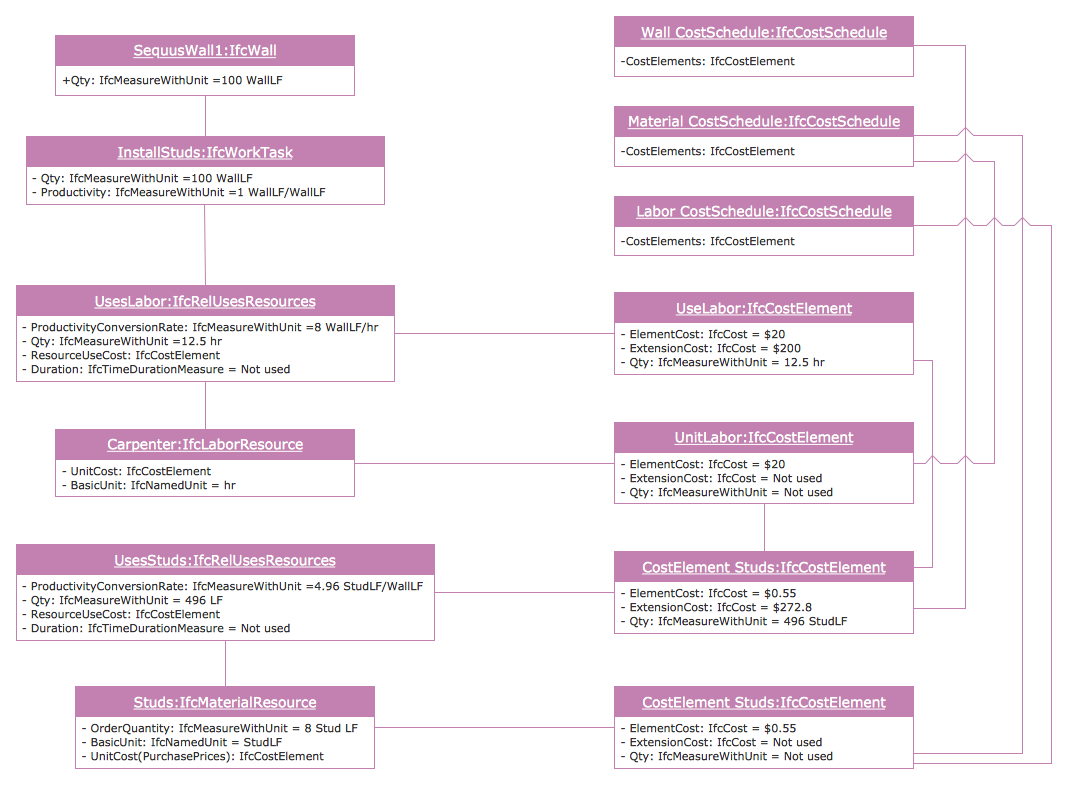## Sample 26: UML Object Diagram — Safety Inspection

Rapid UML sample: UML object diagram — Safety inspection.

This example is created using ConceptDraw DIAGRAM diagramming software enhanced with Rapid UML solution from ConceptDraw Solution Park.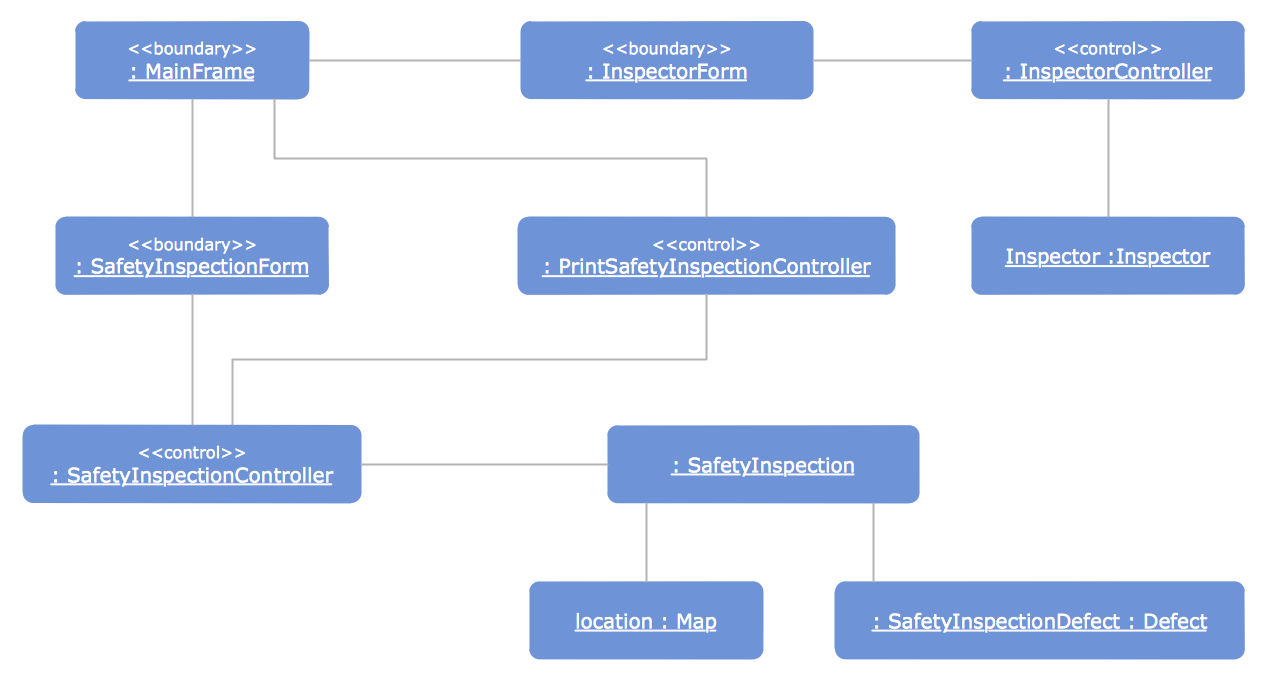## Sample 27: UML Package Diagram — Business Process

Rapid UML sample: UML package diagram — Business process.

This example is created using ConceptDraw DIAGRAM diagramming software enhanced with Rapid UML solution from ConceptDraw Solution Park.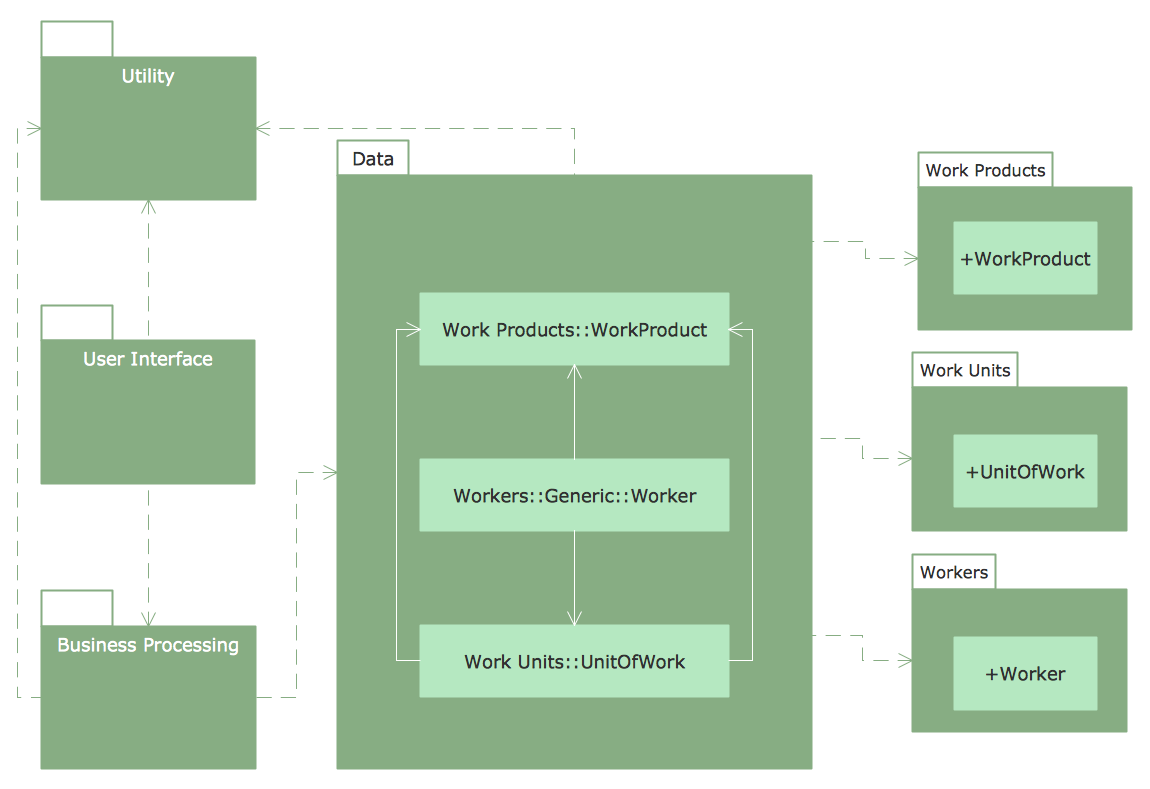## Sample 28: UML Sequence Diagram

Rapid UML sample: UML sequence diagram.

This example is created using ConceptDraw DIAGRAM diagramming software enhanced with Rapid UML solution from ConceptDraw Solution Park.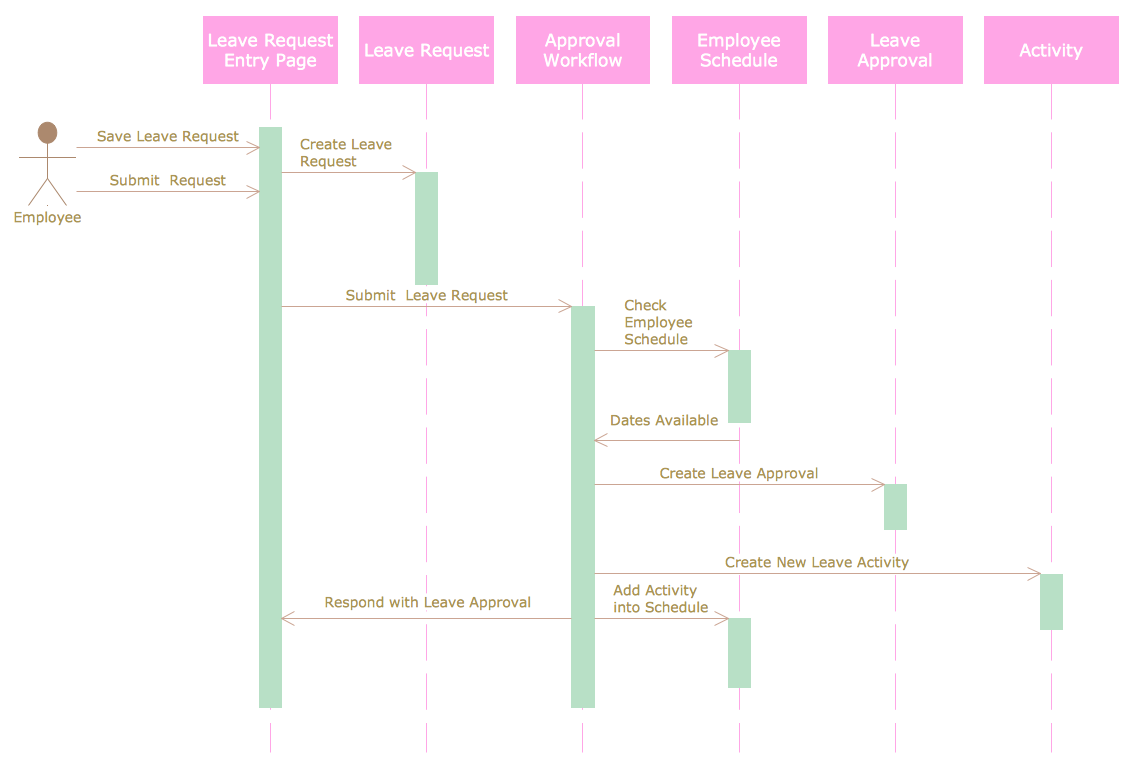## Sample 29: UML Sequence Diagram — GET Request

Rapid UML sample: UML sequence diagram — GET request.

This example is created using ConceptDraw DIAGRAM diagramming software enhanced with Rapid UML solution from ConceptDraw Solution Park.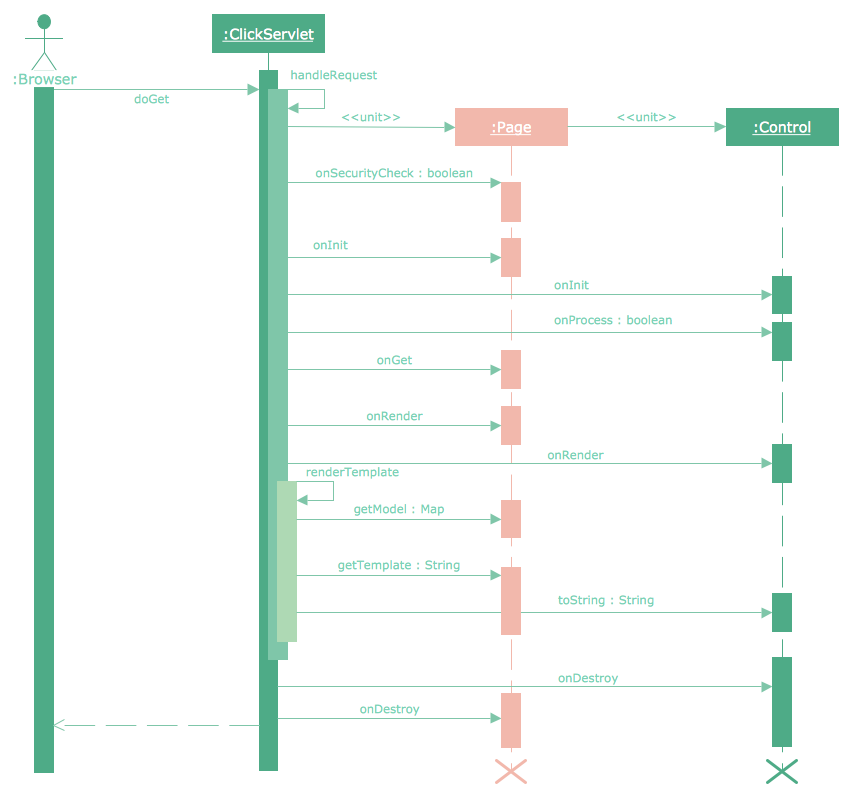## Sample 30: UML Sequence Diagram — Help Desk

Rapid UML sample: UML sequence diagram — Help desk.

This example is created using ConceptDraw DIAGRAM diagramming software enhanced with Rapid UML solution from ConceptDraw Solution Park.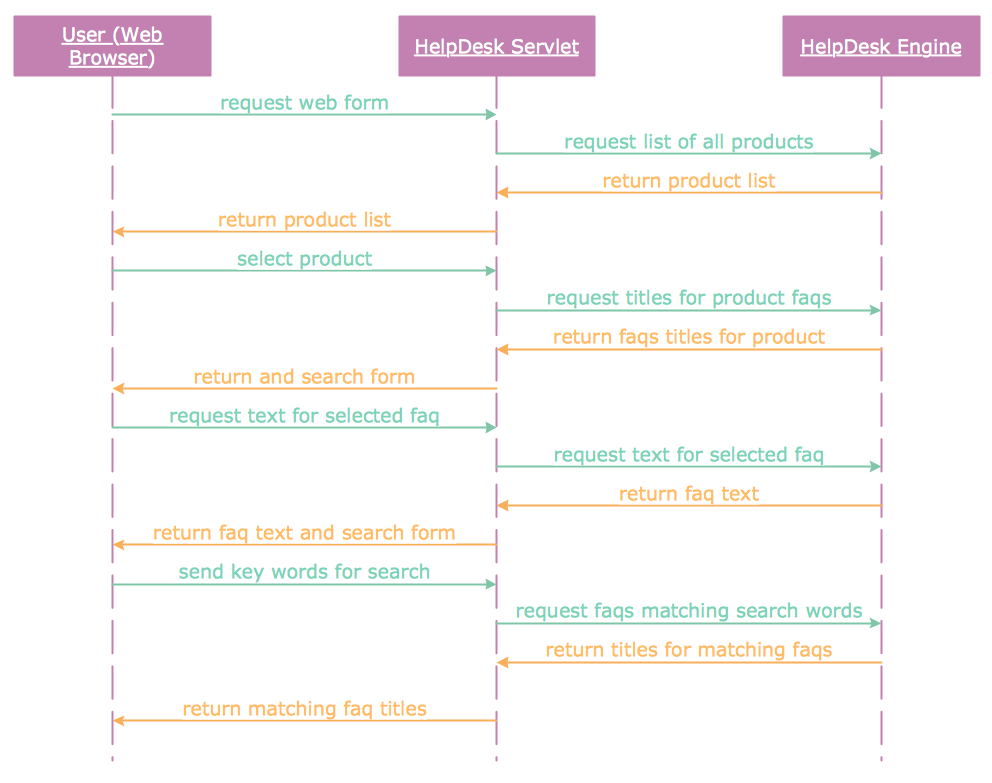## Sample 31: UML Sequence Diagram — Ticket Processing System

Rapid UML sample: UML sequence diagram — Ticket processing system.

This example is created using ConceptDraw DIAGRAM diagramming software enhanced with Rapid UML solution from ConceptDraw Solution Park.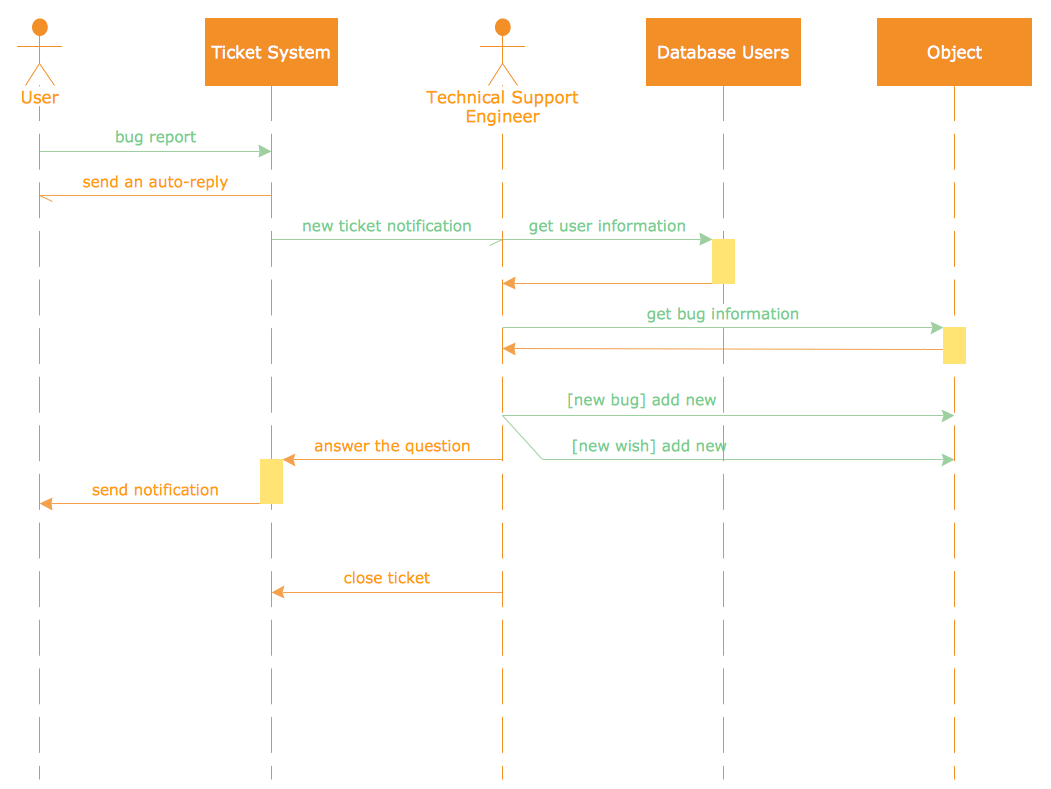## Sample 32: UML State Machine Diagram — State Transitions of RT-component

Rapid UML sample: UML state machine diagram — State transitions of RT-component.

This example is created using ConceptDraw DIAGRAM diagramming software enhanced with Rapid UML solution from ConceptDraw Solution Park.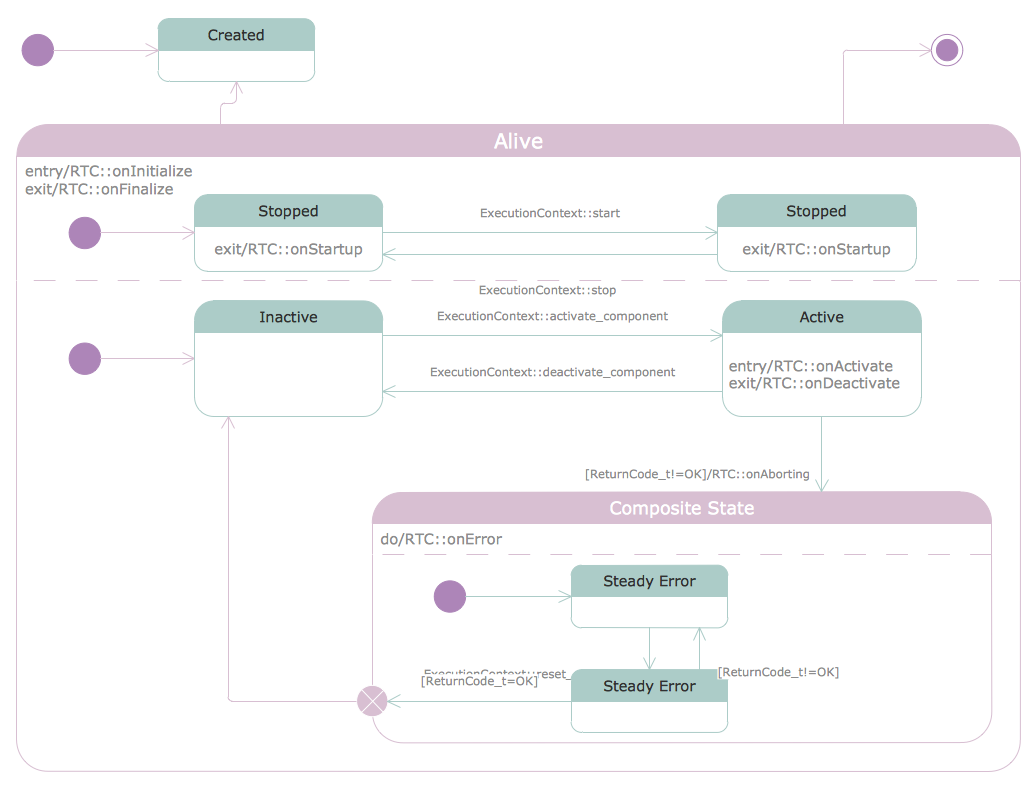## Sample 33: UML Timing Diagram

Rapid UML sample: UML timing diagram.

This example is created using ConceptDraw DIAGRAM diagramming software enhanced with Rapid UML solution from ConceptDraw Solution Park.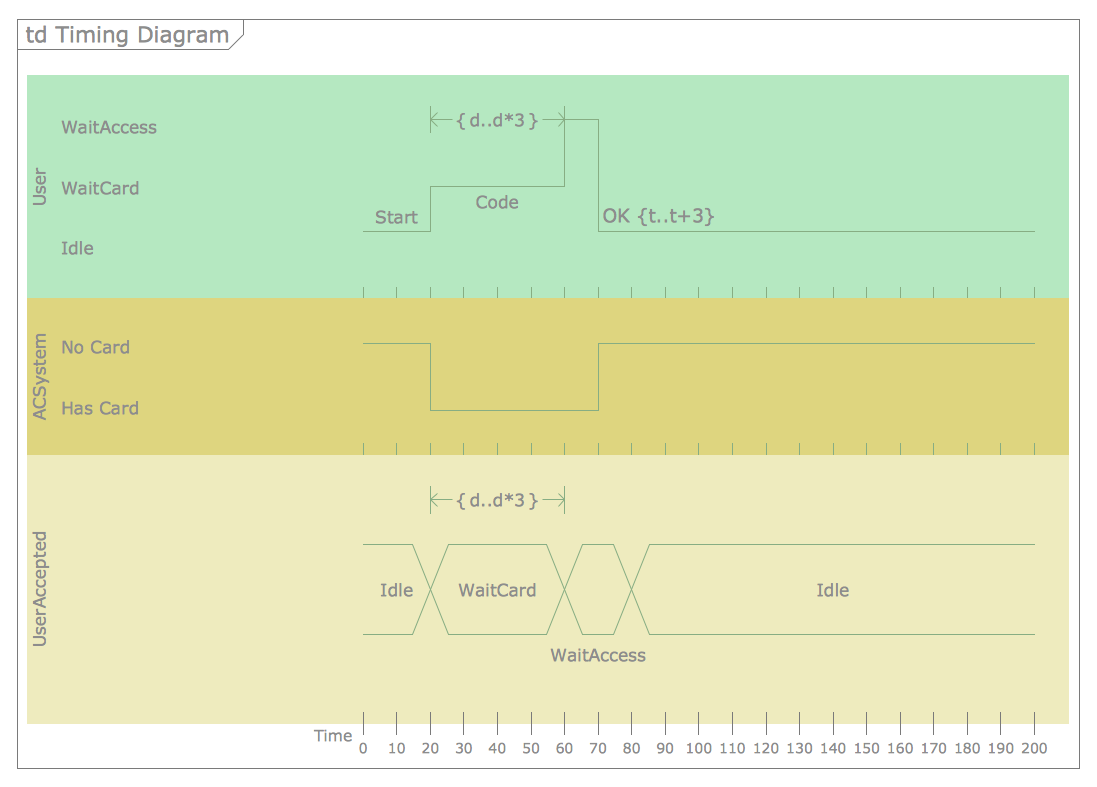## Sample 34: UML Use Case Diagram — Project Administrator

Rapid UML sample: UML use case diagram — Project administrator.

This example is created using ConceptDraw DIAGRAM diagramming software enhanced with Rapid UML solution from ConceptDraw Solution Park.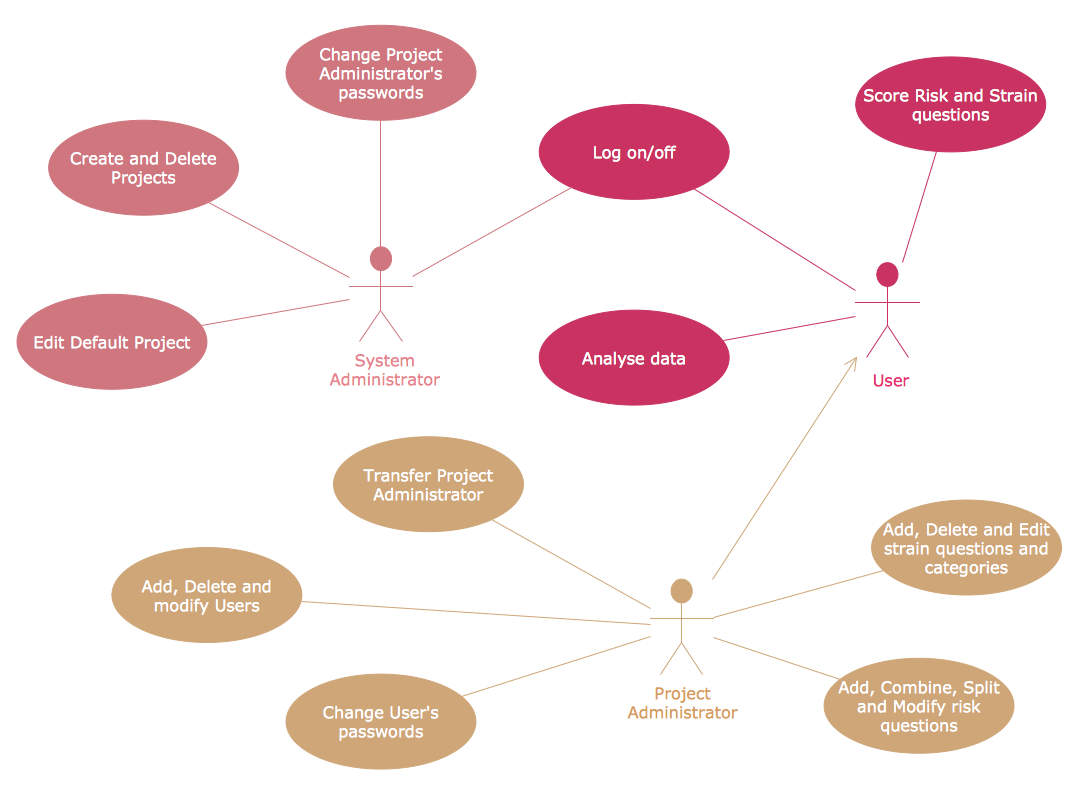## Sample 35: UML Use Case Diagram — Ticket Processing System

Rapid UML sample: UML use case diagram — Ticket processing system.

This example is created using ConceptDraw DIAGRAM diagramming software enhanced with Rapid UML solution from ConceptDraw Solution Park.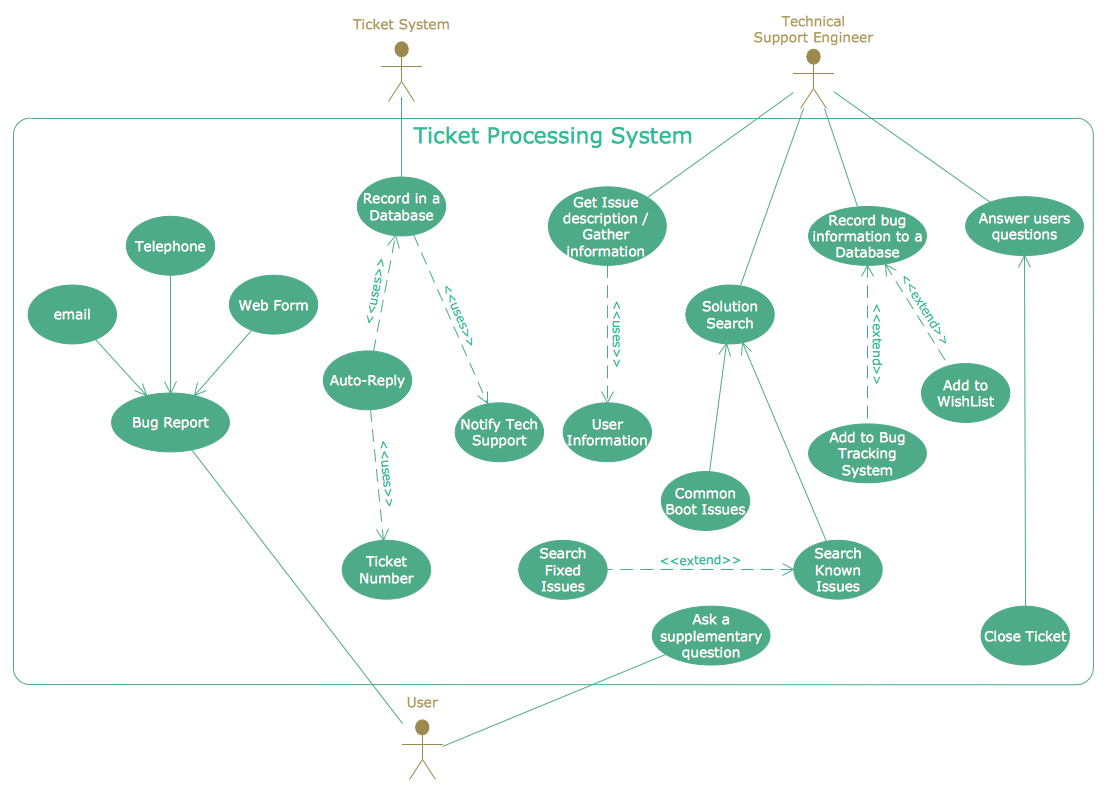## Sample 36: UML Use Case Diagram — Trading System Usage Scenarios

Rapid UML sample: UML use case diagram — Trading system usage scenarios.

This example is created using ConceptDraw DIAGRAM diagramming software enhanced with Rapid UML solution from ConceptDraw Solution Park.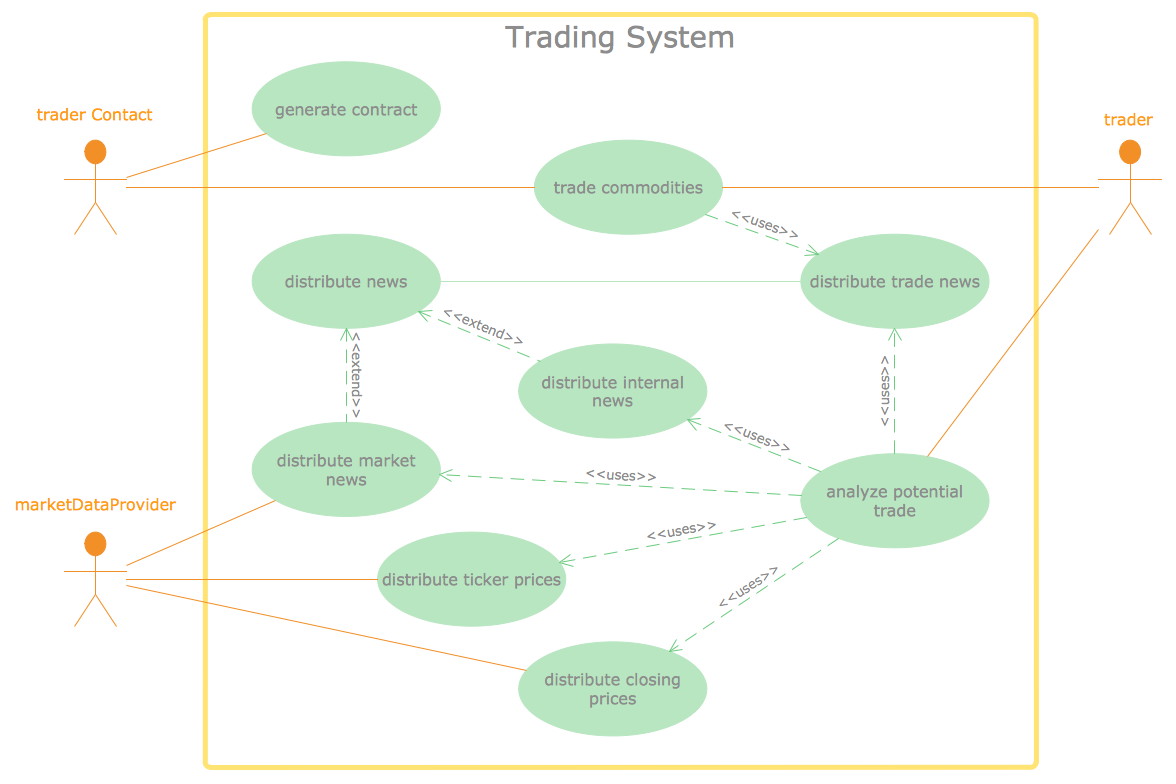## Sample 37: UML Use Case Diagram — System of Goods Selling via Catalogues

Rapid UML sample: UML use case diagram — System of goods selling via catalogues.

This example is created using ConceptDraw DIAGRAM diagramming software enhanced with Rapid UML solution from ConceptDraw Solution Park.